### Home > CAAC > Chapter 1 > Lesson 1.1.3 > Problem1-22

1-22.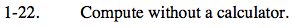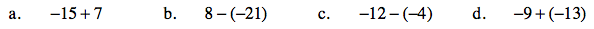One strategy is to draw a diagram and eliminate zeros. Refer to the Math Notes box at right from lesson 1.1.3 to help set up the diagram.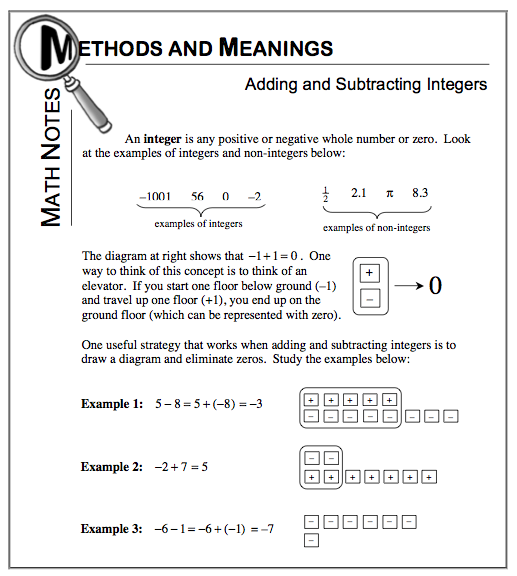−15 + 7 = −8

8 − (−21) = 8 + 21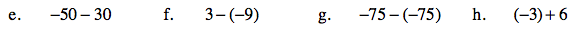Use the strategies shown in problems (a) and (b) to help you solve.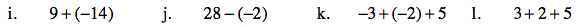Separate the problem into two parts. Solve the first part and then attempt the remaining part of the problem.